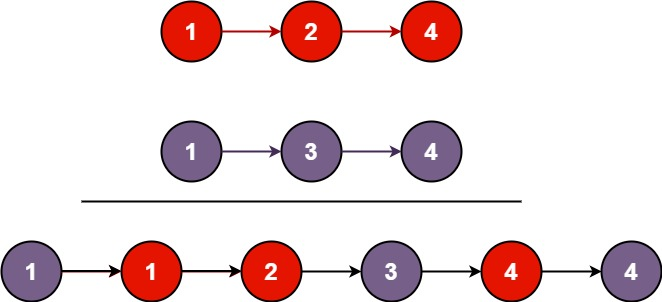| English | 简体中文 |

# 21. Merge Two Sorted Lists

## Description

Merge two sorted linked lists and return it as a sorted list. The list should be made by splicing together the nodes of the first two lists.

Example 1:Input: l1 = [1,2,4], l2 = [1,3,4]
Output: [1,1,2,3,4,4]


Example 2:

Input: l1 = [], l2 = []
Output: []


Example 3:

Input: l1 = [], l2 = 
Output: 


Constraints:

• The number of nodes in both lists is in the range [0, 50].
• -100 <= Node.val <= 100
• Both l1 and l2 are sorted in non-decreasing order.